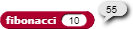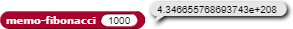# A converter-to-memoized for recursive functions (with any number of parameters)

I created a function that will automatically memoize other (esp. recursive) functions. In other words: users don't need to modify any code by themselves.
Even though it's working now, I consider it still under development, mostly because of metaprogramming complexities. To put it bluntly, the current implementation is a bit messy (but working nonetheless!).

Background
Calculating small values of fibonacci (), i.e. the nth number in the Fibonacci sequence (1, 1, 2, 3, 5, 8, 13, 21, …) is something the canonical (naive) recursive implementation will do pretty fast.For larger numbers, calculating fibonacci () is taking too much time. The processing time is in the order of 2^n, so e.g. fibonacci (31) takes about twice as long to calculate as fibonacci (30). This is caused by the fact that naive fibonacci () has 2 branches of recursion, and the same underlying values are recalculated over and over again.

This is where the memoizer comes in:

It transforms the object function (in this case: fibonacci ()) such that it will reuse values that were already calculated in other branches, drastically cutting processing time: from O(2^n) back to O(n) - in twice the time it takes to calculate memo-fibonacci (30) you can now calculate memo-fibonacci (60).

Thus, calculating even really high numbers within the Fibonacci sequence becomes a breeze:Combined with the Bignums library, showing how big the result actually is:

Project highlights

• a single variable (“lookup tables”) keeps track of any number of function-specific lookup tables, each storing known values of that function with various parameters.
• the memoizer copies the definition of an object function (i.e. a reporter or predicate) to a new function called “memo-“{object function}. E.g. “fibonacci ()” becomes “memo-fibonacci ()”.
• it will prefix some blocks calling a lookup function, which will search the appropriate (if more than one parameter is involved: multi-level) lookup table associated with the new (memo-)function.
• it will modify any REPORT block within the function’s definition, such that it will store calculated values in the lookup table.
• there’s also a function to reset the whole memoizing thing: all of the memoized versions of functions will be deleted, as will all associated lookup tables. I don’t think I will want to keep this reset mechanism in the final version of the project, but for now it’s handy, for debugging purposes.

Next step